# Motor Control ICs Forum Discussions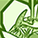Level 2
Level 2# EVAL-M1-101TF configuration with a developped Power PCB (based on EVAL-M1-05F804R)

Hello,

We developped a specific power PCB to drive a Brushless Motor (34KV and 20KW)

Specifications in datasheet are :

No. of Pole : 62

Arms : 3.7A

Rated Speed : 34 RPM/V (I try with 25V)

Line to Line Resistance : 0.008Ohms

Line to Line Inductance : 0.008mH

Configuration file is attached to the post

Here is a video of the motor try to run:  Oscillation_Video

-We put a 500rpm value set. But the motor oscillate between 200 and 600rpm

-The start of the motor is a little bit difficult.

Our schematic is the same than EVAL-M1-05F804R except these values :

-amplification part of the shunt : R18=1KOhms / R30=1220Ohms / R26=5412Ohms.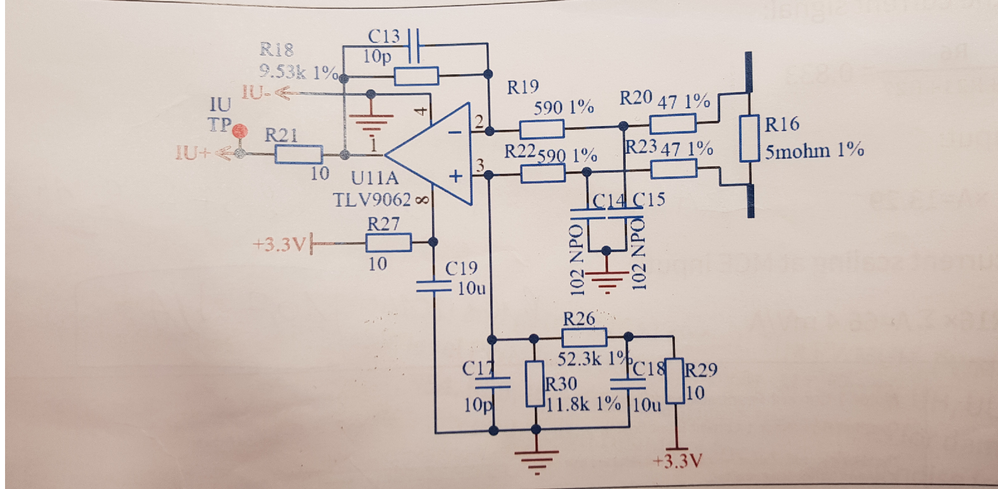Do you have some ideas on how to solve these oscillations behavior ?

Regards,

1 SolutionModeratorModerator# Re: EVAL-M1-101TF configuration with a developped Power PCB (based on EVAL-M1-05F804R)

First of all, you need need to verify that all parameters are correct. I found the following parameter incorrect (Compare your motor parameters with the .mc2 file) :

1. Here is the phase R&L, so it is  half of Line to Line

Line to Line Resistance : 0.008Ohms

Line to Line Inductance : 0.008mH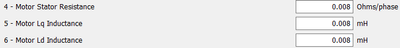These parameters are 0.004Ohms, 0.004mH.

For more motor parameters measure and input, you can refer this manual:how to measure motor parameters

2. For current measurment.   R18=1KOhms / R30=1220Ohms / R26=5412Ohms.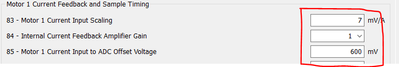OP gain G ≈ (R18+R19+R20)/(R19+R20) = (1000+590+47)/(590+47) =2.57

IU+ voltage: VIU+  = R16*I*G = 12.85mV/A

Voltage offset: Vo = R30/(R26+R29+R30)*3.3 V = 1220/(5412+10+1220) *3.3V = 0.606V

There is offset circuit on IMC101TF board: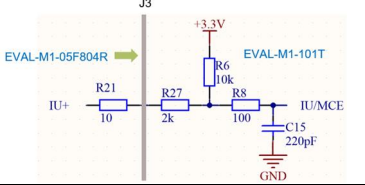So, Current scaling  =  12.85*0.833mV/A = 10.7mV/A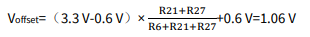These two parameters need modify on your MCEWizard .

The current scaling is very low, and the rated amps is 3.7A, internal current gain is 1. So that the current ADC value is very low, The noise interference will be serious. You can modify the internal gain 1 to 3 or higher. Or modify hardware : Increase OP gain / Increase R shunt.

3. For current measurment adjustment. First you can refer this EVAL-M1-IM535-UserManual  page 13-15, use “VF Diagnostic” function in MCEDesigner. Check whether the current HW and parameters are allright.

4.Use single shunt, You selected Phase Shift mode current sensing, you should tune the Q86 and Q90 values.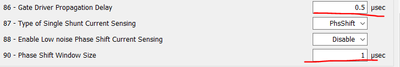For more detail information of current sensing, you should refer Software Reference Manual 2.1.7.2 Single Shunt Current Measurement and4.1 How to check if the current sensing setup is good.

5. For Motor Tuning problems, suggestion refer Chapter 4 of Software Reference Manual  .

2 RepliesModeratorModerator# Re: EVAL-M1-101TF configuration with a developped Power PCB (based on EVAL-M1-05F804R)

First of all, you need need to verify that all parameters are correct. I found the following parameter incorrect (Compare your motor parameters with the .mc2 file) :

1. Here is the phase R&L, so it is  half of Line to Line

Line to Line Resistance : 0.008Ohms

Line to Line Inductance : 0.008mHThese parameters are 0.004Ohms, 0.004mH.

For more motor parameters measure and input, you can refer this manual:how to measure motor parameters

2. For current measurment.   R18=1KOhms / R30=1220Ohms / R26=5412Ohms.OP gain G ≈ (R18+R19+R20)/(R19+R20) = (1000+590+47)/(590+47) =2.57

IU+ voltage: VIU+  = R16*I*G = 12.85mV/A

Voltage offset: Vo = R30/(R26+R29+R30)*3.3 V = 1220/(5412+10+1220) *3.3V = 0.606V

There is offset circuit on IMC101TF board:So, Current scaling  =  12.85*0.833mV/A = 10.7mV/AThese two parameters need modify on your MCEWizard .

The current scaling is very low, and the rated amps is 3.7A, internal current gain is 1. So that the current ADC value is very low, The noise interference will be serious. You can modify the internal gain 1 to 3 or higher. Or modify hardware : Increase OP gain / Increase R shunt.

3. For current measurment adjustment. First you can refer this EVAL-M1-IM535-UserManual  page 13-15, use “VF Diagnostic” function in MCEDesigner. Check whether the current HW and parameters are allright.

4.Use single shunt, You selected Phase Shift mode current sensing, you should tune the Q86 and Q90 values.For more detail information of current sensing, you should refer Software Reference Manual 2.1.7.2 Single Shunt Current Measurement and4.1 How to check if the current sensing setup is good.

5. For Motor Tuning problems, suggestion refer Chapter 4 of Software Reference Manual  .Employee
Employee# Re: EVAL-M1-101TF configuration with a developped Power PCB (based on EVAL-M1-05F804R)